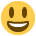#### Howdy, Stranger!

It looks like you're new here. If you want to get involved, click one of these buttons!

Supported by

# OpenSesame 3.1.6 Jazzy James released!

## Help make OpenSesame even better!

First a small promo: You can participate in a usability experiment to make OpenSesame even better by entering the following in the debug window (and restarting OpenSesame):

``````import pip
pip.main(['install', 'opensesame-extension-actpup'])
``````

And now for the new release …

OpenSesame 3.1.6 Jazzy James is the sixth maintenance release in the 3.1 series. This release fixes a regression that was introduced in 3.1.5, which caused a crash when multiple linked `sketchpad`s occurred in the same `sequence`.

If you are upgrading from OpenSesame 3.0 or earlier, please see the list of important changes:

## Bug fixes and improvements

OpenSesame:

• Updated to 3.1.6
• Issue #508: Crash when there are mulitple linked copies of a sketchpad in the same sequence (Bug) (Major)

## Packages (Windows Python 2.7 package)

### Detailed package information

``````name: opensesame_3.1.6-py2.7-win32-1
channels:
- cogsci
- defaults
dependencies:
- python==2.7.12
- anaconda-client=1.4.0=py27_0
- backports=1.0=py27_0
- backports_abc=0.4=py27_0
- bzip2=1.0.6=vc9_3
- clyent=1.2.2=py27_0
- arrow=0.7.0=py_0
- humanize=0.5.1=py_0
- oauthlib=1.0.3=py_0
- psychopy=1.82.01=py27_0
- pygame=1.9.2a0=py27_0
- pyglet=1.2.4=py27_0
- python-datamatrix=0.4.4=py_0 # updated in 3.1.5
- python-fileinspector=1.0.2=py_0 # updated in 3.1.3
- python-opensesame=3.1.6=py_0 # updated in 3.1.6
- python-pseudorandom=0.2.2=py27_0
- python-pygaze=0.6.0a21=py_0 # Updated in 3.1.3
- python-qdatamatrix=0.1.13=py_0 # updated in 3.1.5
- python-qnotifications=1.1.1=py_0 # updated in 3.1.3
- python-qosf=1.1.8=py_0
- python-qprogedit=4.0.9=py_0 # updated in 3.1.5
- qscintilla2=2.9.1=py27_vc9_0
- requests-oauthlib=0.6.1=py_0
- webcolors=1.5=py27_0
- configparser=3.5.0b2=py27_1
- decorator=4.0.10=py27_0
- entrypoints=0.2.2=py27_0
- freetype=2.5.5=vc9_1
- functools32=3.2.3.2=py27_0
- get_terminal_size=1.0.0=py27_0
- ipykernel=4.3.1=py27_0
- ipython=4.2.0=py27_0
- ipython_genutils=0.1.0=py27_0
- ipywidgets=4.1.1=py27_0
- jinja2=2.8=py27_1
- jpeg=8d=vc9_0
- jsonschema=2.5.1=py27_0
- jupyter=1.0.0=py27_3
- jupyter_client=4.3.0=py27_0
- jupyter_console=4.1.1=py27_0
- jupyter_core=4.1.0=py27_0
- libpng=1.6.22=vc9_0
- libtiff=4.0.6=vc9_2
- markdown=2.6.6=py27_0
- markupsafe=0.23=py27_2
- mistune=0.7.2=py27_0
- mkl=11.3.3=1
- nbconvert=4.2.0=py27_0
- nbformat=4.0.1=py27_0
- notebook=4.2.1=py27_0
- numpy=1.11.1=py27_0
- openssl=1.0.2h=vc9_0
- path.py=8.2.1=py27_0
- pickleshare=0.5=py27_0
- pillow=3.2.0=py27_1
- pip=8.1.2=py27_0
- pyflakes=1.2.3=py27_0
- pygments=2.1.3=py27_0
- pyopengl=3.1.1a1=np111py27_0
- pyopengl-accelerate=3.1.1a1=np111py27_0
- pyqt=4.11.4=py27_6
- pyserial=2.7=py27_0
- python=2.7.12=0
- python-dateutil=2.5.3=py27_0
- pytz=2016.4=py27_0
- pyyaml=3.11=py27_4
- pyzmq=15.2.0=py27_0
- qt=4.8.7=vc9_8
- qtawesome=0.3.3=py27_0
- qtconsole=4.2.1=py27_0
- qtpy=1.0.2=py27_0
- requests=2.10.0=py27_0
- scipy=0.17.1=np111py27_1
- setuptools=23.0.0=py27_0
- simplegeneric=0.8.1=py27_1
- singledispatch=3.4.0.3=py27_0
- sip=4.16.9=py27_2
- six=1.10.0=py27_0
- sqlite=3.13.0=vc9_1
- ssl_match_hostname=3.4.0.2=py27_1
- traitlets=4.2.1=py27_0
- vs2008_runtime=9.00.30729.1=2
- wheel=0.29.0=py27_0
- yaml=0.1.6=0
- zlib=1.2.8=vc9_3
- pip:
- cffi==1.7.0
- expyriment==0.8.0 # Upgrade manually to 0.8.1.opensesame2
- imageio==1.5
- moviepy==0.2.2.11
- opensesame-extension-osf==1.0.12
- opensesame-plugin-media-player-mpy==0.1.6
- opensesame-windows-launcher==0.4.1
- pycparser==2.14
- python-bidi==0.4.0
- sounddevice==0.3.3
- tqdm==4.7.6
- pyaudio==0.2.9 # Added in 3.1.3
- openpyxl==2.4.0 # Added in 3.1.3
- fastnumbers==1.0.0 # Added in 3.1.5
- prettytable==0.7.2 # Added in 3.1.5
prefix: opensesame_3.1.6-py2.7-win32-1
``````

• Me and some other people as i could see in the forum have a big problem with the newest version. On windows 10, 64bit the installation does not leave a folder anywhere. It is impossible to start OpenSesame because it does not appear within your installed programs (Nor anywhere else for that matter).

Could anyone help us?

• I noticed those posts, yes. For me OpenSesame install fine on Windows 10, and I'm not sure what it could be.

On windows 10, 64bit the installation does not leave a folder anywhere.

If find that unlikely, at least if you don't get an error message. I think it's more likely that it's copied to an unusual location. If you run the installer and click on the Details button, you will see all files being copied, and (among loads of stuff), you can also see where they are copied to. Does that help? And what if you explicitly specify a location to install to?

As a workaround, you can also download the standalone zip package, unzip it, and just run it from there. There is no difference in the software.

Anyway, i can use it now but i thought you should know